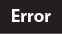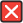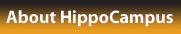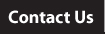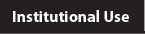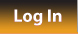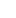More OER Help Center Log In About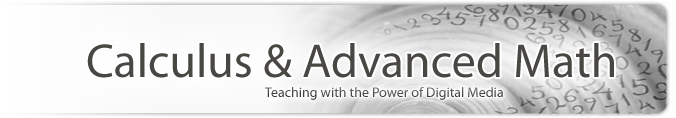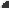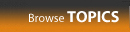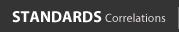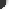Select All ContentPresentations
NROC Collection
Developmental Math - Trigonometry
Phoenix College Collection
Trigonometry
Calculus I
Calculus II
Calculus III
Linear Algebra
Differential Equations
Why U Collection
Infinite SeriesWorked Examples
NROC Collection
Developmental Math - Trigonometry
Trigonometry
Pre-Calculus
Calculus
Differential Equations
Linear Algebra
Phoenix College Collection
Trigonometry
Calculus I
Calculus II
Calculus III
Linear Algebra
Differential Equations
Nursing MathSimulations
NROC Collection
Developmental Math - Trigonometry
PhET Collection
Calculus
Trigonometry
Linear Algebra
 Search:Select an item from the left column to display a list of Topics. (To select multiple items, hold down the command or control key.)
Sequential
Topic Name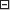hide column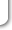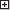maximize
Open Textbook to Accompany Developmental Math (English version):
Libro de Texto Abierto to Accompany Developmental Math (Spanish version):
Adult Ed Hippo Playlists (for TABE and GED) developed by the State of Kentucky:
The Basics of Rational Expressions:
Logarithms:
Tips for Taking Derivatives:
Basic Calculus Refresher:
MIT BLOSSOMS:
What is a Function?: